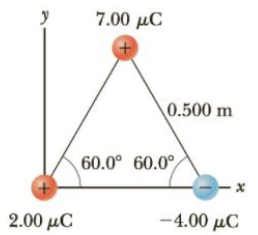Chapter 15, Problem 13P

Chapter
Section
Textbook Problem

Three point charges are located at the corners of an equilateral triangle as in Figure P15.13. Find the magnitude and direction of the net electric force on the 2.00 μC charge.Figure P15.13 Problems 13 and 24.

To determine
The magnitude and direction of net electric force on 2μC .

Explanation

The force diagram is given by,

In the above diagram,

• F1 is the force due to q1 on q2 .
• F3 is the force due to q3 on q2 .

Formula to calculate the force due to q1 on q2 is,

F1=keq1q2a2      (I)

• ke is the Coulomb constant.
• a is the side of the triangle

Formula to calculate the force due to q3 on q2 is,

F3=keq3q2a2       (II)

Net force along the x direction is,

Fx=F3F1cos60ο       (III)

Net force along the y direction is,

Fy=F1sin60ο       (IV)

Formula to calculate the magnitude of net electric force is,

FR=Fx2+Fy2       (V)

Substitute Equations (III) and (IV) in (V).

FR=(keq3q2a2(keq1q2a2)cos60ο)2+(keq1q2a2sin60ο)2=(keq2a2)(q3q1cos60ο)2+(q1sin60ο)2

Substitute 8.99×109N.m2/C2 for ke , 7.00μC for q1 , 2.00μC for q2 , 4.00μC for q3 and 0.500 m for a.

FR={[(8.99×109N.m2/C2)(2.00μC)(0.500m)2]×((4

Still sussing out bartleby?

Check out a sample textbook solution.

See a sample solution

The Solution to Your Study Problems

Bartleby provides explanations to thousands of textbook problems written by our experts, many with advanced degrees!

Get Started